# Fredholm solvability

(diff) ← Older revision | Latest revision (diff) | Newer revision → (diff)

Letbe a real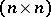-matrix anda vector.

The Fredholm alternative instates that the equation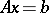has a solution if and only iffor every vector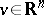satisfying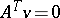.

This alternative has many applications, e.g. in bifurcation theory. It can be generalized to abstract spaces. So, letandbe Banach spaces (cf. Banach space) and letbe a continuous linear operator. Let, respectively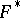, denote the topological dual of, respectively, and let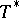denote the adjoint of(cf. also Duality; Adjoint operator). Define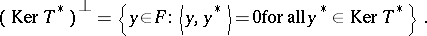An equationis said to be normally solvable (in the sense of F. Hausdorff) if it has a solution whenever(cf. also Normal solvability). A classical result states that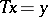is normally solvable if and only if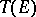is closed in.

In non-linear analysis, this latter result is used as definition of normal solvability for non-linear operators.

The phrase "Fredholm solvability" refers to results and techniques for solving differential and integral equations via the Fredholm alternative and, more generally, Fredholm-type properties of the operator involved.

How to Cite This Entry:
Fredholm solvability. Encyclopedia of Mathematics. URL: http://encyclopediaofmath.org/index.php?title=Fredholm_solvability&oldid=14350
This article was adapted from an original article by G. IsacThemistocles M. Rassias (originator), which appeared in Encyclopedia of Mathematics - ISBN 1402006098. See original article